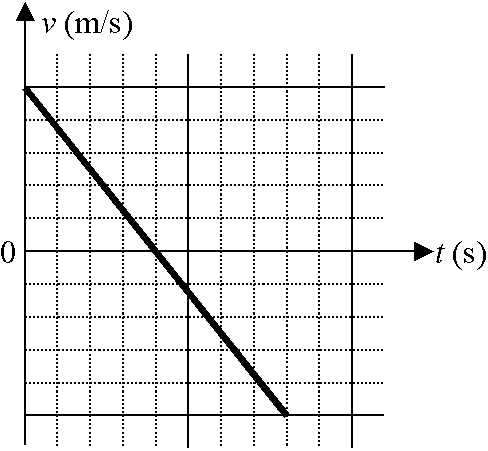# Basic Kinematics, 1st year physics

asheik234
1. Problems:

A ball is launched vertically upward and returns to ground level 8 seconds later. Let upward be the positive direction.

To what maximum height does the ball rise?

-------------------------------------------------------------------------------------A remote-controlled car's velocity is graphed as a function of time as shown. On this graph, the vertical axis is divided into 4 m/s ticks and the horizontal axis is divided into 5 second ticks.

What is the car's greatest displacement from its initial position?

-------------------------------------------------------------------------------------
A speeding motorist travels with a constant speed of 20 m/s on a straight road. At time t = 0, he passes a hidden police car which is at rest. The instant that the motorist passes, the police car begins accelerating at a constant rate of 5 m/s2 in order to catch the motorist.

How much distance has the police car traveled when it reaches the motorist?
-------------------------------------------------------------------------------------

A ball is dropped from rest at the top of a 480 meter tall building. The ball freely falls with constant acceleration for 4 seconds; at this time, the ball reaches a terminal velocity.

(a) How far has the ball fallen when it reaches terminal velocity?

(b) What is the terminal velocity of the ball?

(c) What is the total time that the ball takes to reach the ground?

---------------------------------------------------------------------------------------
A rocket fires from rest with an upward acceleration of 20 m/s2 for 2 seconds. After this time, the engine shuts off and the rocket freely falls back to the surface of the Earth.

Determine the amount of time the rocket is in the air.

## Homework Equations

v= v(naught) + at

x = x(naught) + v(naught)t + (1/2)at^2

v^2 = v(naught)^2 + 2a (x-x(naught))

## The Attempt at a Solution

On q1, they don't give me initial or final velocity, that's why I'm stuck.

q2, I did all my calculations and got -800, which is wrong.

q3, 40m/s * 8s would be 320m, but that's wrong

q4, I don't know how to calculate terminal velocity, an explanation would be helpful

Homework Helper
In Q1, you can find the initial and final velocities using your first formula.
Show your calc for Q2 and we'll see where the error is.
Q3 has a constant speed portion plus an accelerated motion portion. You will need to do two separate calcs and add the distances.

Terminal velocity is a constant speed, reached when the force of air resistance equals and cancels out the force of gravity. Work with the constant acceleration for 4 seconds to find the distance and speed reached.

azizlwl

asheik234
In Q1, you can find the initial and final velocities using your first formula.
Show your calc for Q2 and we'll see where the error is.
Q3 has a constant speed portion plus an accelerated motion portion. You will need to do two separate calcs and add the distances.

Terminal velocity is a constant speed, reached when the force of air resistance equals and cancels out the force of gravity. Work with the constant acceleration for 4 seconds to find the distance and speed reached.

For q2, I took the final position and added it to the initial position, now that I think about it, wouldn't it be 1600m, instead of 800m. Can you verify for me, Thanks

q3, I don't get it, the cop car is trying to accelerate in order to catch up to the motorcar, how is there a constant speed portion?

q4, still confused, how do I calculate how far the ball has fallen, would I use g=10m/s2, and multiply it by 4, and after that how would i calculate the constant speed and time.

q5, an explanation would be helpful again

majormaaz
---------------------------------------------------------------------------------------
A rocket fires from rest with an upward acceleration of 20 m/s2 for 2 seconds. After this time, the engine shuts off and the rocket freely falls back to the surface of the Earth.

Determine the amount of time the rocket is in the air.

## Homework Equations

v= v(naught) + at

x = x(naught) + v(naught)t + (1/2)at^2

v^2 = v(naught)^2 + 2a (x-x(naught))

okay, let's split this problem into two parts: When the rocket goes up, and when it comes back down.
When it goes up, it takes 2 seconds. Bam, done. But, you want to use your first equation to find out the final velocity
plug in your values, and you'll get V

Now, we're going to see how long it takes for the rocket to fall back
Since equation #3 doesn't have time, we'll use #2, but we need the distance it'll fall.
so...we model the rocket going up by using Eq #3 (the rocket travels the same distance going up and down/assuming your teacher doesn't believe in warp holes)
plug in the same values you used for your first equation and you should get the distance traveled.

Now, use your third equation, rearrange for time, and solve, using V ( I boldfaced it) as your V naught, distance and gravity.

azizlwl
You make the calculation as simple as possible that you can calculate in your head.
But you can't get a correct answer.
Can you draw a velocity vs. time graph if you throw an object upward with v m/s and displacement vs. time too.
From these graphs you can see the statistic of the object moving at a constant acceleration.
Surely for a simple constant velocity, velocity vs. time and displacement vs. time must be easy.

Formula-hunting not the only strategies to solve physics problems.

Last edited: